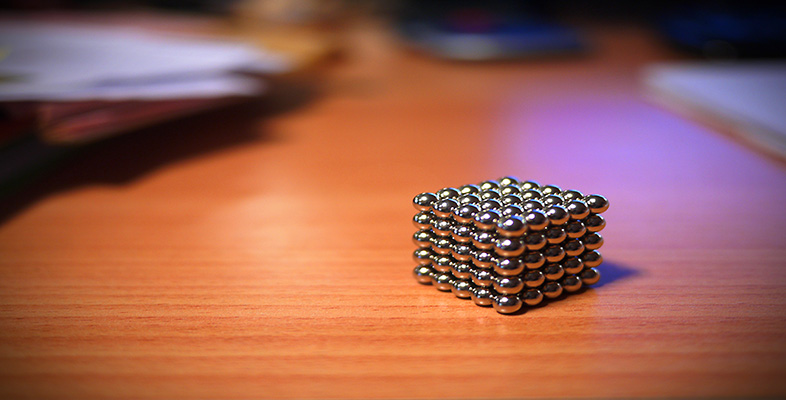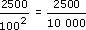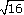Science, Maths & Technology

### Become an OU studentSquares, roots and powers

Start this free course now. Just create an account and sign in. Enrol and complete the course for a free statement of participation or digital badge if available.

# 1.1.1 Try some yourself

## Activity 1

Evaluate the following:

• (a) 62

• (b) 0.52

• (c) 1.52

• (a) 62 = 6 × 6 = 36

• (b) 0.52 = 0.5 × 0.5 = 0.25

• (c) 1.52 = 1.5 × 1.5 = 2.25

## Activity 2

Square carpet tiles measure 50 cm by 50 cm (or 0.5 m by 0.5 m).

• (a) What area is covered by one tile? Give your answer (i) in square centimetres (cm2) and (ii) in square metres (m2).

• (b) What area, in m2, will 100 tiles cover?

• (c) How many tiles are needed to cover 1 square metre?

• (d) How many tiles are needed to cover a room with an area of 16 m2?

• (e) If the room in (d) is square, how many tiles go along each edge?

• (a)(i) One tile covers 50 × 50 = 2500 cm2.

• (a)(ii) To convert this to m2, you could divide by 1002 to give= 0.25 m2.

• Alternatively (and more simply) convert first: each tile measures

• 0.5 m × 0.5 m and so has an area 0.5 m × 0.5 m = 0.25 m2.

• (b) One tile covers 0.25 m2 so 100 tiles cover 100 × 0.25 = 25 m2.

• (c) Since each tile covers 0.25 m2, 4 tiles will cover 4 × 0.25 = 1 m2.

• (d) 4 tiles cover 1 m2 so 16 × 4 = 64 tiles cover 16 m2.

• (e) If the room is square and the area is 16 m2 then each edge must be 4 m, since 4 × 4 = 16 (or=4). So 8 tiles go along each edge.

• (You could also have deduced this from your answer to (d), since 64 tiles is 8 × 8 tiles.)